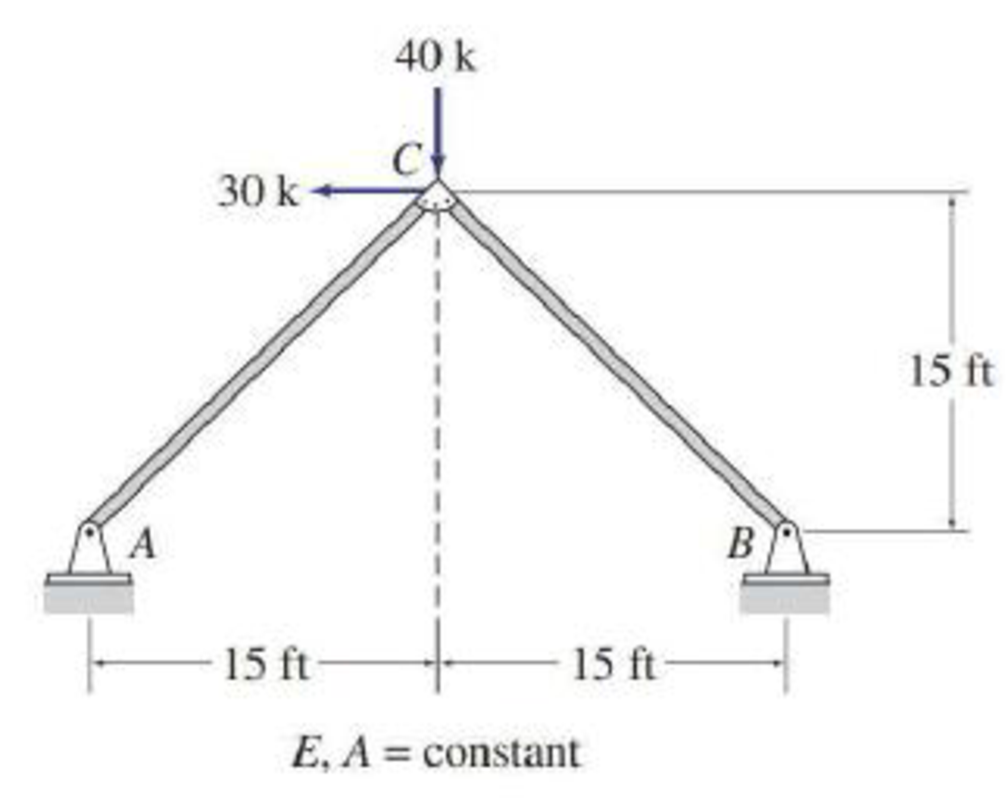# Determine the force in each member of the trusses shown in Figs. P10.1–P10.5 by utilizing structural symmetry. FIG. P10.1, P10.16

#### Solutions

Chapter
Section
Chapter 10, Problem 16P
Textbook Problem
27 views

## Determine the force in each member of the trusses shown in Figs. P10.1–P10.5 by utilizing structural symmetry.FIG. P10.1, P10.16

To determine

Find the force in each member of the truss using structural symmetry.

### Explanation of Solution

Given information:

The structure is given in the Figure.

The young’s modulus E and area A is constant.

Apply the sign conventions for calculating reactions, forces, and moments using the three equations of equilibrium as shown below.

• For summation of forces along x-direction is equal to zero (Fx=0), consider the forces acting towards right side as positive (+) and the forces acting towards left side as negative ().
• For summation of forces along y-direction is equal to zero (Fy=0), consider the upward force as positive (+) and the downward force as negative ().
• For summation of moment about a point is equal to zero (Matapoint=0), consider the clockwise moment as negative and the counter clockwise moment as positive.

Method of joints:

The negative value of force in any member indicates compression (C) and the positive value of force in any member indicates tension (T).

Calculation:

Refer the given structure.

The structure is symmetric with respect to the s axis.

Sketch the half loading for the given structure as shown in Figure 1.

Sketch the substructure with symmetric boundary conditions as shown in Figure 5.

Find the reactions and member end forces of substructure using equilibrium equations and to the left of s axis.

The member end forces to the right of s axis are obtained by the reflection.

Summation of moments about A is equal to 0.

MA=020×15Cx×15=0Cx=20k

Summation of forces along y-direction is equal to 0.

+Fy=0Ay20=0Ay=20k

Summation of forces along x-direction is equal to 0.

+Fx=0AxCx=0Ax=20k

Consider joint A, find the force in the member AC:

+Fy=020+FACsin(45°)=0FAC=28

### Still sussing out bartleby?

Check out a sample textbook solution.

See a sample solution

#### The Solution to Your Study Problems

Bartleby provides explanations to thousands of textbook problems written by our experts, many with advanced degrees!

Get Started

Find more solutions based on key concepts
Using a sound meter, the following measurements were made for the following sources: rock band at a concert (10...

Engineering Fundamentals: An Introduction to Engineering (MindTap Course List)

What is an InfoSec blueprint?

Management Of Information Security

A(n) _____ report consolidates data usually with totals, tables, or graphs. (558) a. detailed b. summary c. exc...

Enhanced Discovering Computers 2017 (Shelly Cashman Series) (MindTap Course List)

What is competitive disadvantage? Why has it emerged as a factor?

Principles of Information Security (MindTap Course List)

5. Identify the main components of an information system. What is a mission-critical system?

Systems Analysis and Design (Shelly Cashman Series) (MindTap Course List)

Identify and briefly discuss five types of operating system user interfaces.

Principles of Information Systems (MindTap Course List)

Calculate the reactions at the built-in support at C, neglecting the weights of the members.

International Edition---engineering Mechanics: Statics, 4th Edition

What semi-precision tool is used to take angular measurements?

Precision Machining Technology (MindTap Course List)

Convert 5 megawatts of power into BTU/hr, ft-lbs/s, and kJ/hr.

Fundamentals of Chemical Engineering Thermodynamics (MindTap Course List)

Which form of SHA was developed by private designers?

Network+ Guide to Networks (MindTap Course List)

If your motherboard supports ECC DDR3 memory, can you substitute non-ECC DDR3 memory?

A+ Guide to Hardware (Standalone Book) (MindTap Course List)